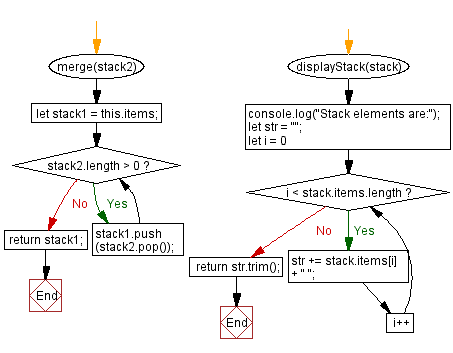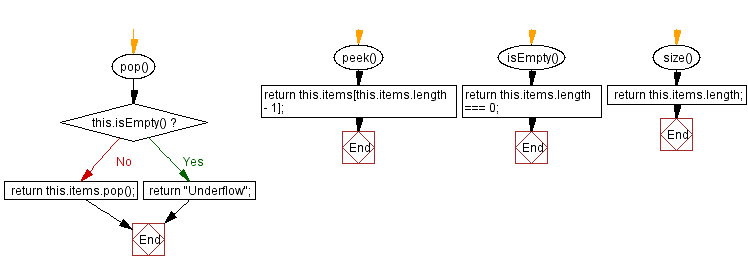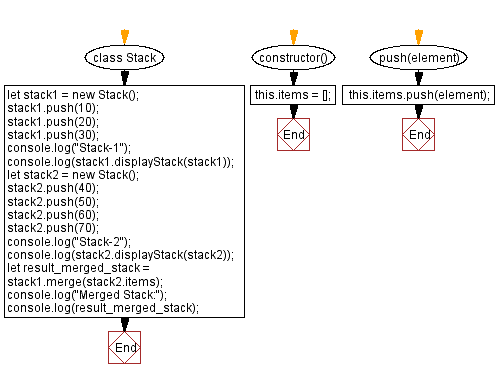# JavaScript Exercises: Merge two stacks into one

## JavaScript Stack: Exercise-20 with Solution

Write a JavaScript program to merge two stacks into one.

Sample Solution:

JavaScript Code:

``````class Stack {
constructor() {
this.items = [];
}

push(element) {
this.items.push(element);
}
pop() {
if (this.isEmpty()) {
return "Underflow";
}
return this.items.pop();
}
peek() {
return this.items[this.items.length - 1];
}
isEmpty() {
return this.items.length === 0;
}
size() {
return this.items.length;
}

merge(stack2) {
let stack1 = this.items;
while (stack2.length > 0) {
stack1.push(stack2.pop());
}
return stack1;
}

displayStack(stack) {
console.log("Stack elements are:");
let str = "";
for (let i = 0; i < stack.items.length; i++)
str += stack.items[i] + " ";
return str.trim();
}
}
let stack1 = new Stack();
stack1.push(10);
stack1.push(20);
stack1.push(30);
console.log("Stack-1");
console.log(stack1.displayStack(stack1));
let stack2 = new Stack();
stack2.push(40);
stack2.push(50);
stack2.push(60);
stack2.push(70);
console.log("Stack-2");
console.log(stack2.displayStack(stack2));
let result_merged_stack = stack1.merge(stack2.items);
console.log("Merged Stack:");
console.log(result_merged_stack);
```
```

Sample Output:

```Stack-1
Stack elements are:
10 20 30
Stack-2
Stack elements are:
40 50 60 70
Merged Stack:
[10,20,30,70,60,50,40]
```

Flowchart:Live Demo:

See the Pen javascript-stack-exercise-20 by w3resource (@w3resource) on CodePen.

Improve this sample solution and post your code through Disqus

Stack Previous: Move the nth element from the bottom of the stack to the top.
Stack Exercises Next: Implement a stack using a linked list.

What is the difficulty level of this exercise?

Test your Programming skills with w3resource's quiz.

﻿